International
Tables for
Crystallography
Volume A1
Symmetry relations between space groups
Edited by Hans Wondratschek and Ulrich Müller

International Tables for Crystallography (2011). Vol. A1, ch. 1.6, pp. 52-54

## Section 1.6.5. Handling cell transformations

Ulrich Müllera*

aFachbereich Chemie, Philipps-Universität, D-35032 Marburg, Germany
Correspondence e-mail: mueller@chemie.uni-marburg.de

### 1.6.5. Handling cell transformations

| top | pdf |

It is important to keep track of the coordinate transformations in a sequence of group–subgroup relations. A Bärnighausen tree can only be correct if every atomic position of every hettotype can be derived from the corresponding positions of the aristo­type. The mathematical tools are described in Sections 1.2.2.3, 1.2.2.4and 1.2.2.7of this volume and in Part 5of Volume A.

A basis transformation and an origin shift are mentioned in the middle of a group–subgroup arrow. This is a shorthand notation for the matrix–column pair (Seitz symbol; Section 1.2.2.3) or the 4 × 4 matrix (augmented matrix, Section 1.2.2.4) to be used to calculate the basis vectors, origin shift and coordinates of a maximal subgroup from those of the preceding space group. If there is a sequence of several transformations, the overall changes can be calculated by multiplication of the 4 × 4 matrices.

Let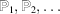be the 4 × 4 matrices expressing the basis vector and origin changes of several consecutive cell transformations.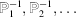are the corresponding inverse matrices. Let a, b, c be the starting (old) basis vectors and,,be the (new) basis vectors after the consecutive transformations. Letandbe the augmented columns of the atomic coordinates before and after the transformations. Then the following relations hold: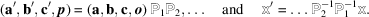is the vector of the origin shift, i.e. its components are the coordinates of the origin of the new cell expressed in the coordinate system of the old cell;is a zero vector. Note that the inverse matrices have to be multiplied in the reverse order.

#### Example 1.6.5.1

Take a transformation from a cubic to a rhombohedral unit cell (hexagonal setting) combined with an origin shift of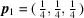(in the cubic coordinate system), followed by transformation to a monoclinic cell with a second origin shift of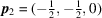(in the hexagonal coordinate system). From Fig. 1.6.5.1(left) we can deduce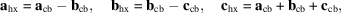which in matrix notation is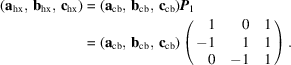In the fourth column of the 4 × 4 matrix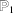we include the components of the origin shift,: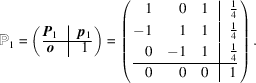The inverse matrixcan be calculated by matrix inversion, but it is more straightforward to deduce it from Fig. 1.6.5.1; it is the matrix that converts the hexagonal basis vectors back to the cubic basis vectors: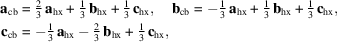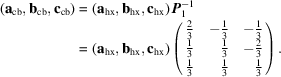The column part (fourth column) of the inverse matrix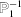is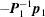(cf. equation 1.2.2.6):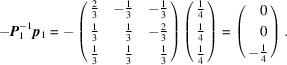Therefore,is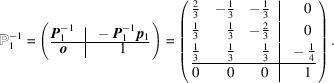Similarly, for the second (hexagonal to monoclinic) transformation, we deduce the matrices,and the column part of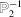,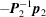, from the right image of Fig. 1.6.5.1: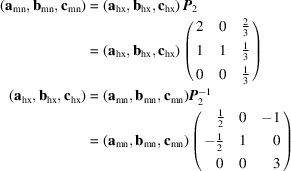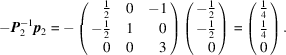For the basis vectors of the two consecutive transformations we calculate: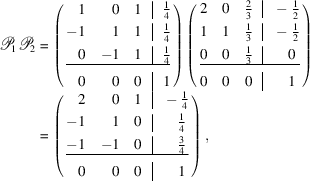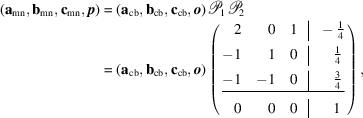which corresponds to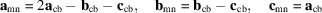and an origin shift of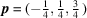in the cubic coordinate system. The corresponding (cubic to monoclinic) coordinate transformations result from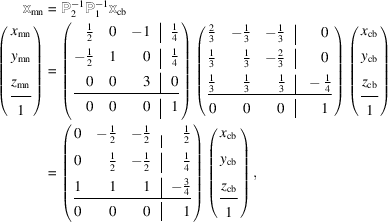which is the same as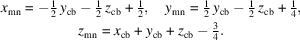Figure 1.6.5.1 | top | pdf |Relative orientations of a cubic to a rhombohedral cell (hexagonal setting) (left) and of a rhombohedral cell (hexagonal setting) to a monoclinic cell (the monoclinic cell has an acute angle β).